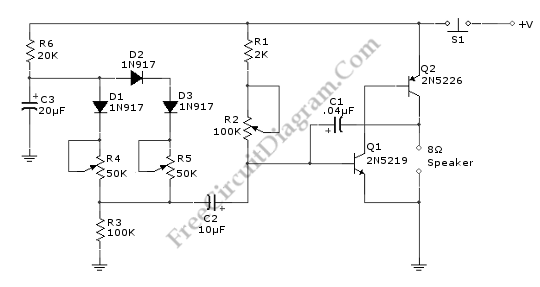# Discrete Sliding Tone (Frequency Ramp) DoorbellThis doorbell circuit produces a low tone that will slide up to higher frequency. The equivalent total resistance connected between the base of Q1 and ground (Rbg) , and coupling capacitor  C1  determines the AF oscillator’s frequency. The resistance (Rbg) is equal to (R2+R1)R3.  Here is the schematic diagram of the circuit:The R2 is used to set the initial bias condition, adjusted to produce a pleasant low starting frequency doorbell tone. D1 will start to conduct when Capacitor C3 charge through R6 until it reaches D1 bias  voltage level. Then the value of Rbg is paralleled by R4 and D1, and R5-D2-D3, and the values of diode’s equivalent resistance is gradually decreased as the C3 voltage ramp up.  This decreasing resistance value make the output tone slides up in frequency.  Two different diode path is provided to extend the linear area of diode conduction transition slope. With two path with different biases, after the single diode path has saturated, the second path provide further linear increase at higher voltage level.